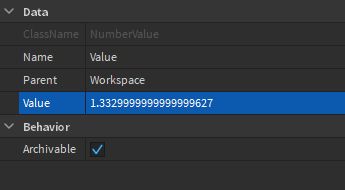# How do I truncate a NumberValue properly?

I’ve been trying to round off my NumberValue with so many kind of scripts yet many don’t work for some reason - actually they kinda do except it finishes off with random numbers at the very end. I heard it is a rounding off error? Is there a method around this?

``````-- I did not use them all at the same time, just a list of methods I've tried
-- First
script.Parent.Value = tonumber(string.sub(tostring(script.Parent.Value * 100), 1, 4)) / 100

--- Second
local str = "%.2f"
script.Parent.Value = string.format(str, script.Parent.Value)

-- Third
function round(num,place)
return math.floor(num/place+0.5)*place
end
round(script.Parent.Value,0.01)
``````

All did not work, well kinda - what it gave was from NumberValue.Value = 1.3329999999999999627
into NumberValue.Value = 1.3300000000000000711

(I only want 2 decimal places)

you could do this:

``````function Round(number)
local num = string.split(tostring(number), ".")

if #num >= 2 then
local var = string.sub(num, 1, 2)
num = num.."."..var
end
return num
end

Round(1.358908349686)
``````
1 Like

Here is a possible solution

``````
function Round(OrigNum,Place)
assert(Place >= 1,"Invalid Place Given")
local Offset1 = 10^Place
local Offset2 = 10^-Place
local NewNum = math.floor(OrigNum*Offset1)  * Offset2
return NewNum
end
``````

This works because math.floor rounds it down but if we keep the amount of digits out of the rounding then revert the change we would have the digits back

1 Like

Unfortunately, from both answers above, the result was 1.3329999999999999627~

Are you sure you copied the code correctly as it works perfectly on my end.
https://gyazo.com/99c3678e8ee8f2b4c235c05b3e65fb54

I think the issue is with the `*place`, because the issue with floats (number values) is that there are often weird rounding errors. You should perform the necessary calculations, and then floor that value.

``````return math.floor((num/place+0.5)*place)
``````

Here’s the issue, when you print it out, it works but it doesn’t work onto NumberValue.Value. That’s my issue if my post didn’t clarifyThis is because of floating point math. There is a topic that discusses it here. This shouldn’t have any large effect on your game, and it shows you the computer’s interpretation of the value. If you were set a numberValue to 0.4 and print it, you would get 0.4. Using stringvalues can work, but over time that can create errors with math, so unless this math isn’t too necessary I would personally just leave it, as players aren’t going to see the numbervalue’s value, and if they are, using `string.format("%.2f", Value)` or; like scripton said in the topic: using string values and tonumber to solve this.

Simple rounding function that makes sure you don’t get something like 4.00 if you input a 4:

``````local function Round(number)
if math.floor(number) ~= number then
return string.format("%.2f", number)
end
end
``````
1 Like

Please search DevForum first before posting. Inaccurate NumberValue seems to have been a bug for a while.

At no point was this ever a bug. Base ten numbers don’t get along with base two numbers all the time.
Just like how 1/3 is .3333333 etc. One-third can’t be perfectly represented in bases two or ten (and many others

One-tenth can be written as .1 in base ten, but in base two it doesn’t have a clean representation. The closest you can get with a float is 0.100000001490116119384765625

If you want to mess around and learn about the floating point format, check this out.
https://www.h-schmidt.net/FloatConverter/IEEE754.html

2 Likes

So it’s more like a system limitation than a bug.

2 Likes